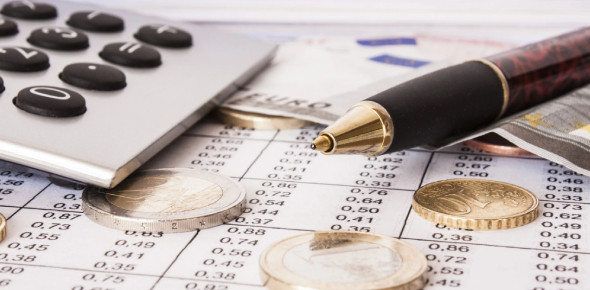# A Quiz To Test Your Accounting Knowledge!

19 Questions | Total Attempts: 227Settings.

• 1.
Current liabilities are:
• A.

Due and receivable within one year

• B.

Due and to be paid out of current assets within one year

• C.

Due, but not payable for more than one year

• D.

Payable if a possible subsequent event occurs

• 2.
Which of the following would most likely be classified as a current liability?
• A.

Two-yr notes payable

• B.

Bonds payable

• C.

Mortgage payable

• D.

Unearned rent

• 3.
When a 30,000, 90-day 5% interest-bearing note payable matures, total payment will amount to
• A.

31,500

• B.

1,500

• C.

30,375

• D.

375

• 4.
The current portion of a long term debt should:
• A.

Be classsified as a long term liability

• B.

Not be separated from the long term portion of debt

• C.

Be paid immediately

• D.

Be reclassified as a current liability

• 5.
The amount of federal income taxes withheld from an employee's goes pay is recorded as an:
• A.

Payroll expense

• B.

Contra account

• C.

Asset

• D.

Liability

• 6.
Land acquired so it can be resold in the future is listed in the balance sheet as an
• A.

Fixed asset

• B.

Current asset

• C.

Investment

• D.

Intangible asset

• 7.
Factors contributing to a decline in the usefulness of a fixed asset may be divided into the following:
• A.

Salvage and functional

• B.

Physical and functional

• C.

Residual and salvage

• D.

Functional and residual

• 8.
A fixed asset's estimated value at the time it is to be retired from service.
• A.

Book value

• B.

Residual value

• C.

Market value

• D.

Carrying value

• 9.
All of the following below are need for the celebration of straight-line depreciation except:
• A.

Cost

• B.

Residual value

• C.

Estimated life

• D.

Units produced

• 10.
The method of determining depreciation that yields successive reductions in the periodic depreciation charge over the estimated life of the asset is
• A.

Units-of-production

• B.

Declining-balance

• C.

Straight- line

• D.

Time-valuation

• 11.
Current liabilities are:
• A.

Due and receivable within one year

• B.

Due and to be paid out of current assets within one year

• C.

Due, but not payable for more than one year

• D.

Payable if a possible subsequent event occurs

• 12.
Which of the following would most likely be classified as a current liability?
• A.

Two-yr notes payable

• B.

Bonds payable

• C.

Mortgage payable

• D.

Unearned rent

• 13.
When a \$30,000, 90-day, 5% interest-bearing note payable matures, total payment will amount to:
• A.

31,500

• B.

1,500

• C.

30,375

• D.

375

• 14.
The current portion of long term debt should:
• A.

Be classified as a long term liability

• B.

Not be separated from the long-term portion of debt

• C.

Be paid immediately

• D.

Be reclassified as a current liability

• 15.
The formula for depreciable cost is:
• A.

Initial cost + residual value

• B.

Initial cost - residual value

• C.

Initial cost - accumulated depreciation

• D.

Depreciable cost = initial cost

• 16.
Expected useful life is:
• A.

Calculated when the asset is sold

• B.

Estimated at the time that the asset is placed in service

• C.

Determined each year that the depreciation calculation is made

• D.

None of the answers are correct

• 17.
The calculation for annual depreciation using the straight-line depreciation method is:
• A.

Initial cost / estimated useful life

• B.

Depreciable cost / estimated useful life

• C.

Depreciable cost * estimated useful life

• D.

Initial cost * estimated useful life

• 18.
The calculation for annual depreciation using the units-of-production method is:
• A.

(initial cost/ estimated output) * the actual year output

• B.

(depreciable cost/ yearly output) * estimated output

• C.

Depreciable cost/ yearly output

• D.

(depreciable cost/ estimated output) * the actual yearly output

• 19.
Computer equipment was acquired at the beginning of the year at a cost of \$65,000 that has an estimated residual value of \$3,000 and an estimated useful life of 5 years. determine the 2nd year's depreciation using straight-line depreciation.
• A.

\$26,000

• B.

\$24,800

• C.

\$12,400

• D.

\$13,000

Related TopicsBack to top